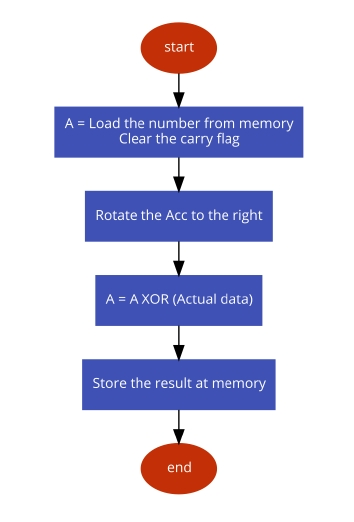# 8085 program to convert an 8 bit number into Grey number

MicrocontrollerMicroprocessor8085

In this program we will see how to find the gray code from an 8-bit number.

### Problem Statement

Write 8085 Assembly language program to convert an 8-bit number stored at 8000H to its equivalent gray code. The result will be stored at 8050H.

### Discussion

In this program we are converting binary to gray code. The procedure is simple. At first we have to shift the content to the right, then perform XOR operation with the sifted content and the actual content. Thus we will get the gray code. For an example if the number is ABH, then the binary value will be (1010 1011), after shifting the value will be (0101 0101) = 55H, now by XORing ABH and 55H, the result will be (1111 1110) = FEH

first input

Data
...
...
8000
AB
...
...

second input

Data
...
...
8000
C9
...
...

third input

Data
...
...
8000
D5
...
...

### Flow Diagram### Program

HEX Codes
Mnemonics
F000
21, 00, 80
LXI H,8000H
F003
7E
MOV A, M
Take the number from memory to Acc
F004
37
STC
Set carry flag
F005
3F
CMC
Complement carry flag
F006
1F
RAR
Rotate right Acc content
F007
AE
XRA M
XOR memory content with A
F008
32, 50, 80
STA 8050H
Store the gray code
F00B
76
HLT
Terminate the program

first input

Data
...
...
8050
FE
...
...

second input

Data
...
...
8050
...
...

third input